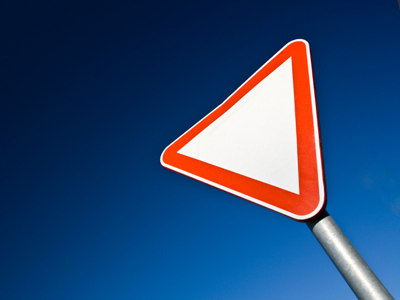The area of a triangle = 12 × base × height.

# Perimeter and Area 2 (Easy)

This is the second of our easy Eleven Plus maths quizzes on perimeter and area. If you have played the first then you will know that the perimeter is the lengths of the sides of a 2D shape added together. The perimeter of a circle is called the circumference. The area of a 2D shape is the ‘space’ enclosed by the shapes perimeter.

To calculate perimeter, circumference and area, you may find these formulae helpful:

• Area of a circle = π × r2
• Circumference of a circle = 2π × r
• Area of rectangle = width x length
• Perimeter of rectangle = (width x 2) + (length x 2)
Have a go at this quiz until you are comfortable with calculating perimeter, area and circumference. Once you’ve learned the rules, you’ll find they aren’t so difficult after all.

1.
A right-angled triangle has a base of 4.8 cm and a height of 6.4 cm. What is its area?
15.36 cm2
28.8 cm2
22.4 cm2
23.04 cm2
Area of a triangle = 12 × base × height
12 x 4.8 x 6.4 = 15.36
2.
A square has a side length of 18 cm. What would be the area of two such squares in mm2?
3,240 mm2
32,400 mm2
6,480 mm2
64,800 mm2
The area of a square = length x length:
18 x 18 = 324. There are two squares so, 324 x 2 = 648.
There are 100 mm2 in one cm2 so 648 x 100 = 64,800
3.
A square has an area of 9.6 cm2. What is its area in mm2?
96 mm2
9,600 mm2
960 mm2
96,000 mm2
There are 100 mm2 in one 1 cm2 so, to convert cm2 to mm2, just multiply by 100
4.
A square has an area of 121 cm2. What is the length of the square’s sides?
12 cm
11 cm
10 cm
9 cm
The area of a square = length × length:
121 = length × length. If you know your times tables you will know that 11 x 11 = 121
5.
A square has an area of 96 cm2. If it is cut from corner to corner into two right-angled triangles, what is the area of each triangle?
24 cm2
36 cm2
48 cm2
96 cm2
Halve the area of the given square: two right angled triangles form a square or a rectangle
6.
A rectangle has an area of 72 cm2. It is 8 cm wide so, what is its length?
11 cm
8 cm
10 cm
9 cm
The area of a rectangle = length × width:
72 cm2 = length x 8:
72 ÷ 8 = 9
7.
A rectangle has a length of 1.2 metres and a width of 1.5 metres. What is its area in cm2?
180 cm2
1,800 cm2
18,000 cm2
180,000 cm2
The area of a rectangle = length x width:
1.2 x 1.5 = 1.8.
There are 10,000 cm2 in one m2 so 1.8 x 10,000 = 18,000
8.
The formula for finding the area of a circle is: Area = π × r2. What is the value of π?
237 or 3.286
227 or 3.142
247 or 3.429
217 or 3
You will find π very useful in maths, so try to remember its value if you can
9.
A circle has a radius of 10 cm. If π = 3.142, what is the area of the circle?
31.42 cm2
314.2 cm2
3,142 cm2
0.3142 cm2
The formula for finding the area of a circle is: Area = π × r2
10.
What is the perimeter of a rectangle of width 6 cm and length 12 cm?
36 cm
72 cm
48 cm
18 cm
Perimeter of a rectangle = 2 × length + 2 × width:
(2 × 6) + (2 × 12) = 12 + 24 = 36 cm.
Do not confuse perimeter and area!
Author:  Frank Evans